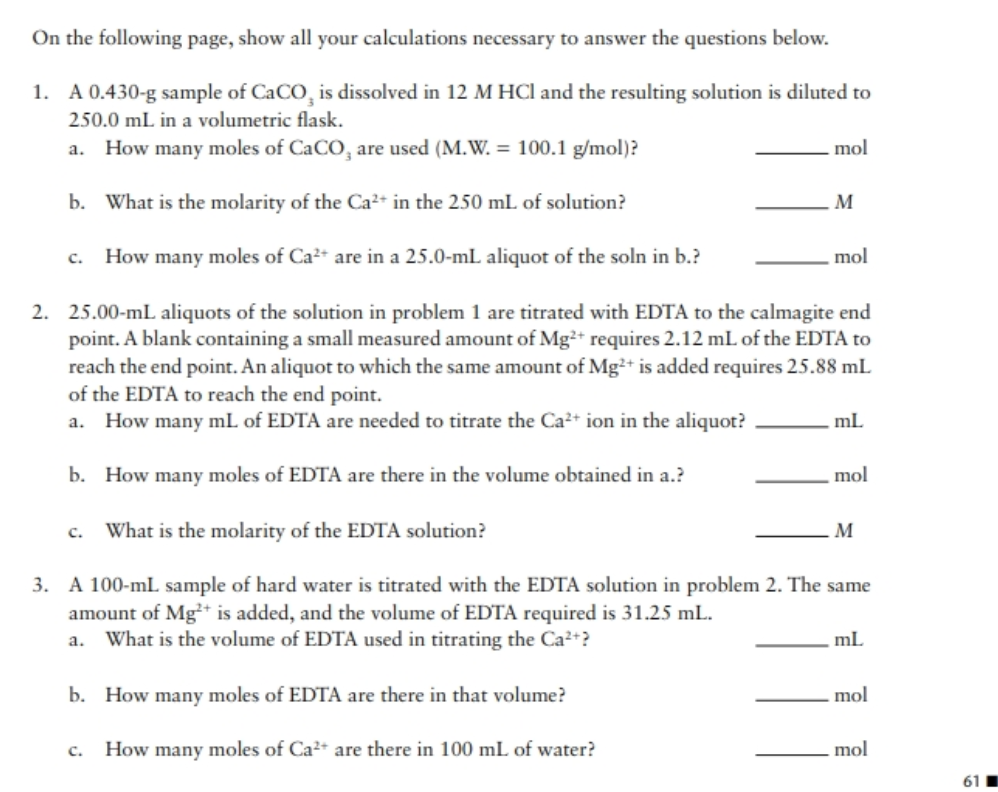# On the following page, show all your calculations necessary to answer the questions below. 1. A 0.430-g sample of CaCO, is dissolved in 12 M HCl and the resulting solution is diluted to 250.0 mL in a volumetric flask. a. How many moles of CaCO, are used (M.W. = 100.1 g/mol)? mol b. What is the molarity of the Ca²+ in the 250 mL of solution? M How many moles of Ca²* are in a 25.0-mL aliquot of the soln in b.? . mol 2. 25.00-mL aliquots of the solution in problem 1 are titrated with EDTA to the calmagite end point. A blank containing a small measured amount of Mg²* requires 2.12 mL of the EDTA to reach the end point. An aliquot to which the same amount of Mg²+ is added requires 25.88 mL of the EDTA to reach the end point. How many mL of EDTA are needed to titrate the Ca²+ ion in the aliquot? ml b. How many moles of EDTA are there in the volume obtained in a.? mol c. What is the molarity of the EDTA solution? M 3. A 100-mL sample of hard water is titrated with the EDTA solution in problem 2. The same amount of Mg* is added, and the volume of EDTA required is 31.25 mL. a. What is the volume of EDTA used in titrating the Ca²+? ml b. How many moles of EDTA are there in that volume? .mol c. How many moles of Ca²+ are there in 100 mL of water? mol

Question

Can you please solve question #2 part a,b,c ? and #3 part a, b, c?help_outlineImage TranscriptioncloseOn the following page, show all your calculations necessary to answer the questions below. 1. A 0.430-g sample of CaCO, is dissolved in 12 M HCl and the resulting solution is diluted to 250.0 mL in a volumetric flask. a. How many moles of CaCO, are used (M.W. = 100.1 g/mol)? mol b. What is the molarity of the Ca²+ in the 250 mL of solution? M How many moles of Ca²* are in a 25.0-mL aliquot of the soln in b.? . mol 2. 25.00-mL aliquots of the solution in problem 1 are titrated with EDTA to the calmagite end point. A blank containing a small measured amount of Mg²* requires 2.12 mL of the EDTA to reach the end point. An aliquot to which the same amount of Mg²+ is added requires 25.88 mL of the EDTA to reach the end point. How many mL of EDTA are needed to titrate the Ca²+ ion in the aliquot? ml b. How many moles of EDTA are there in the volume obtained in a.? mol c. What is the molarity of the EDTA solution? M 3. A 100-mL sample of hard water is titrated with the EDTA solution in problem 2. The same amount of Mg* is added, and the volume of EDTA required is 31.25 mL. a. What is the volume of EDTA used in titrating the Ca²+? ml b. How many moles of EDTA are there in that volume? .mol c. How many moles of Ca²+ are there in 100 mL of water? mol fullscreen

### Want to see this answer and more?

Experts are waiting 24/7 to provide step-by-step solutions in as fast as 30 minutes!*

*Response times may vary by subject and question complexity. Median response time is 34 minutes for paid subscribers and may be longer for promotional offers.
Tagged in
Science
Chemistry

### General Chemistry